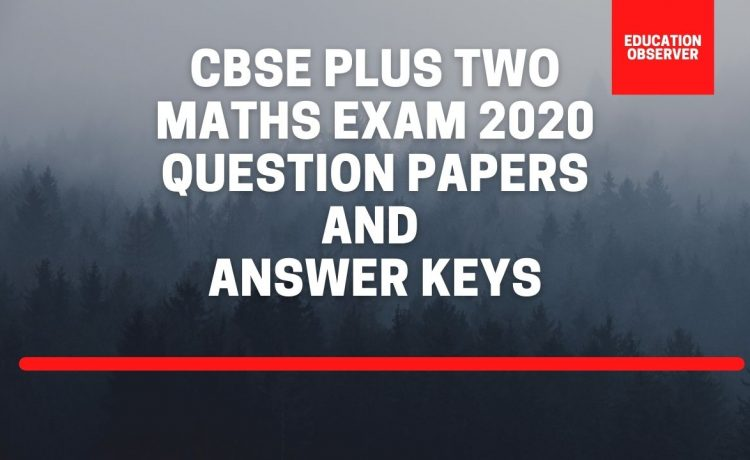# CBSE 12th Maths Question Paper and Solutions of Board Exam 2020Download previous year question papers and answer keys of CBSE Plus Two Maths examination held on March 17, 2020. All set question papers together with their answer keys / marking scheme/ solutions are avaialble for download from the links given below

## CBSE 12th Board Maths Exam 2020

The exam was said to be of moderate difficulty. We are giving question papers and solutions of all the series question papers together with the marking scheme, published by CBSE for the valuation of the answer keys.

### Selected few questions

1. The total revenue received from the sale of x units of a product is given by R(x) = 3x2 + 36x + 5 in rupees. Find the marginal revenue when x = 5, where by marginal revenue we mean the rate of change of total revenue with respect to the number of items sold at an instant.
2. A window is in the form of a rectangle surmounted by a semicircular opening. The total perimeter of the window is 10 metres. Find the dimensions of the window to admit maximum light through the whole opening. How having large windows help us in saving electricity and conserving environment ?
3. Three rotten apples are mixed with seven fresh apples. Find the probability distribution of the number of rotten apples, if three apples are drawn one by one with replacement. Find the mean of the number of rotten apples.
4. In a shop X, 30 tins of ghee of type A and 40 tins of ghee of type B which look alike, are kept for sale. While in shop Y, similar 50 tins of ghee of type A and 60 tins of ghee of type B are there. One tin of ghee is purchased from one of the randomly selected shop and is found to be of type B. Find the probability that it is purchased from shop Y.
5. Find the points on the curve 9y2 = x3, where the normal to the curve makes equal intercepts with both the axes. Also find the equation of the normals.
6. A company manufactures two types of novelty souvenirs made of plywood. Souvenirs of type A requires 5 minutes each for cutting and 10 minutes each for assembling. Souvenirs of type B require 8 minutes each for cutting and 8 minutes each for assembling. Given that total time for cutting is 3 hours 20 minutes and for assembling 4 hours. The profit for type A souvenir is 100 each and for type B souvenir, profit is Rs. 120 each. How many souvenirs of each type should the company manufacture in order to maximize the profit ? Formulate the problem as an LPP and solve it graphically.
7. A company produces two types of goods, A and B, that require gold and silver. Each unit of type A requires 3 g of silver and 1 g of gold while that of B requires 1 g of silver and 2 g of gold. The company can use atmost 9 g of silver and 8 g of gold. If each unit of type A brings a profit of Rs. 40 and that of type B Rs. 50, find the number of units of each type that the company should produce to maximize the profit. Formulate and solve graphically the LPP and find the maximum profit.
8. Two groups are competing for the positions of the Board of Directors of a corporation. The probabilities that the first and second groups will win are 0.6 and 0.4 respectively. Further, if the first group wins, the probability of introducing a new product is 0.7 and the corresponding probability is 0.3 if the second group wins. Find the probability that the new product introduced was by the second group.

The complete set of Mathematics question papers and answer keys of CBSE Plus Two examination 2020 of all the 5 variants of the question papers can be downloaded from the links given below. Both the question papers and keys are available in printable pdf format.

•Tharaka shilpa says:
•mary d'souza says:
•Ravi hegde says: